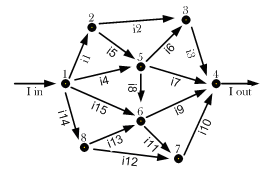1. Engineering
2. Electrical Engineering
3. in the figure the voltages are defined as v121 2...

# Question: in the figure the voltages are defined as v121 2...

###### Question detailsIn the figure, the voltages are defined as V12=φ1- φ2, V46= φ4- φ6, (and more generally: Vmn = φm- φn), where φ1, φ2,... φm are the potentials of the nodes 1,2,...m.
If V21= 1.1 V, V35= 2.5 V, V25= 1.9 V, V16= 8.5 V, what is the voltage V56?

###### Solution by an expert tutor